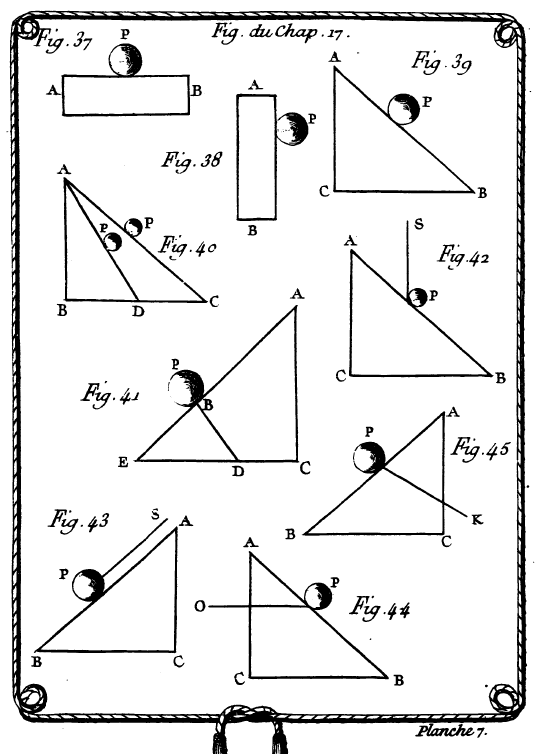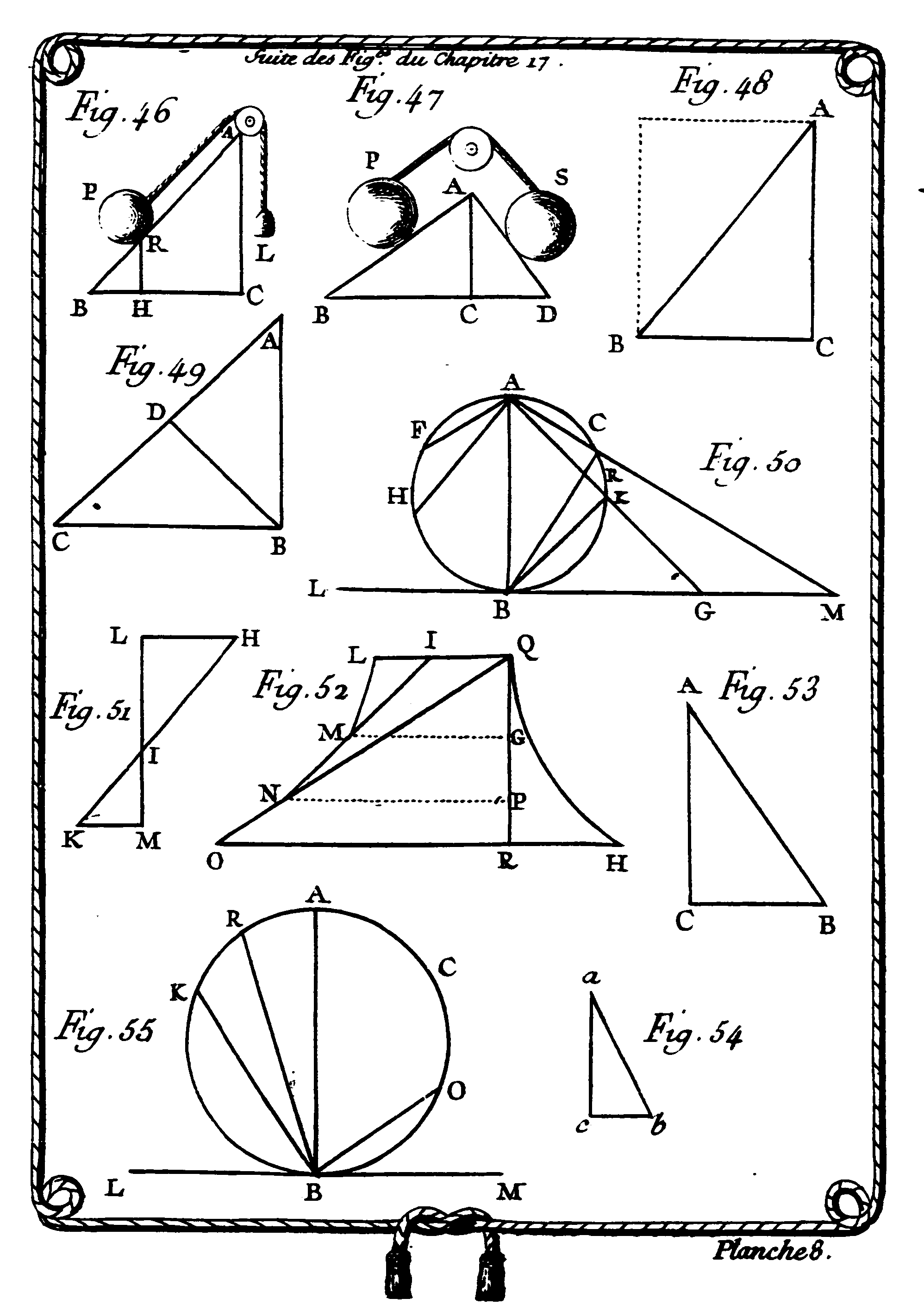Chapter 17. Of Rest, and the Fall of Bodies on an Inclined PlaneChapter 17 is about the fall of the bodies along an inclined plane. According to the gravitational law, the action of gravity is always uniform, and always directed perpendicularly toward the center of the earth. Now, what happens when a body that is falling toward the earth changes its direction or its motion? Du Châtelet argues that there must necessarily be some foreign cause that is combined with the action of gravity upon it. “Foreign” cause means an external cause. In a Newtonian framework it is “impressed force.” Gravity is an example of such a kind of force. Newton has characterized impressed force as active force that causes the change of a body’s state in motion or rest. Inertial force, on the other hand, was a passive and internal principle that is the cause for the body’s attempt to continue in its state. (Note: Seen from this perspective, inertial motion was not a forceless and uncaused motion. Today, inertia is no longer viewed as an inherent force (either motive or resistive), but only equates to inertial mass. Inertial mass is a measure of a body’s resistance to acceleration.)  Du Châtelet maintains that “foreign” causes can be active or passive; the active causes are those that imprint a new motion on the bodies, such as when I throw a stone that would otherwise have fallen had it been acted upon only by the force of its gravity. The passive causes are those that do not imprint any new motion on the body, but that change only its direction (InstPhy, § 401). (Note: From today’s perspective this is wrong, because if an object changes direction it is accelerating. Thus, any change of direction is a change of motion, i.e. acceleration.)

An inclined plane is a plane that makes an oblique angle with the horizontal plane (InstPhy, § 402). Its geometry is based on a right triangle. Given the trianlge ABC {Fig. 39}, then the line AC, perpendicular to the horizontal plane, is called the height of the plane. The line AB, oblique to the horizontal plane, is called the length of the plane. The line BC, that is parallel to the horizontal plane, is called the base of the plane, and the angle ABC, that the plane AB makes with the horizontal plane, is called the angle of inclination of this plane (InstPhy, §§ 403-405).We know that the force of gravity acting on an object is directed through this center of gravity and toward the center of the Earth. The task of determining the net force acting upon an object on an inclined plane presupposes that the force of gravity has been replaced by two components – a parallel and a perpendicular component of force. Du Châtelet concludes that on an inclined plane the relative gravity is to its absolute gravity as the height of the plane to its length (InstPhy, § 407; Fig. 40):

The respective gravity of a body on an inclined plane is to its absolute gravity as the length of the plane is to the height; for this plane does not oppose the perpendicular descent of the body, and consequently does not diminish its absolute gravity except insofar as it is inclined to the horizontal plane, since if it was perpendicular to it, it would not be opposed to it at all […]. Therefore, the more this plane is inclined to the horizontal plane, or (which is the same thing) the less high it is, the more the body is supported by the plane, and consequently the less respective gravity it has; therefore the respective gravity of this body on this plane is to its absolute gravity as the height of the plane is to its length. The respective gravity of the same body on planes that are differently inclined is as the angle of inclination of these planes, for the more this angle increases or decreases, the greater or lesser accordingly is the respective gravity of this body. Thus, the respective gravity of body P is greater on plane AD than on plan AC, for angle ADB is greater than angle ACB. (Copyright © 2018 KB)

To sum up: The respective gravities of the same body on different inclined planes are to each other as the lines of the angle of inclination. Now, consider a vertical plane: the respective gravity degenerates into absolute. Consider a horizontal plane: the respective gravity vanishes (InstPhy, §§ 408-409). From today’s perspective we would explain this situation as follows: There are always at least two forces acting upon any object that is positioned on an inclined plane (assumed to be friction-free): the force of gravity and the normal force. The force of gravity (also known as weight) acts in a downward direction. It can be represented by a vector directed down (along the line the object would fall if it were dropped). When this object is resting on a level surface, its weight acts perpendicularly to the surface and will be equal to the normal force, which is the force keeping the object from falling through the table. The normal force is always perpendicular to the surface.

In §§ 411-416, Du Châtelet eplains under which conditions a body can be kept in equilibrium on an inclined plane (InstPhy, § 411, Fig. 41):

A body that remains at rest on an inclined plane is kept in equilibrium by two powers that counterbalance its absolute gravity. 1. The resistance of the plane, which acts along the line BD perpendicular to this plane; for the weight P presses on the plane along this line, and so the plane presses on the weight in the same direction, because of the equality of action and reaction. 2. The exterior force that supports this body, on the plane. (Copyright © 2018 KB)

As well known, gravity is a force that acts downwards. So, the amount of work one has to do is dependent on the angle the trajectory makes with gravity. When moving up you are pushing yourself in the opposite direction of the force of gravity. Therefore, a positive work is done, which is approximately mgh. This is the reason why it is more difficult to go up a mountain than to travel along a plain (InstPhys, § 418).

The idea of taking the product of weight and distance moved in unit time necessarily implies understanding and acceptance of Galileo’s principle, altough the concept of mechanical work was still in a state of flux. This becomes obvious in this chapter, too. Du Châtelet was aware of Galileo’s discovery that an object rolling down an inclined plane would re-ascend to the same height it had started from, if one were subsequently to let it roll up an inclined plane of the same height, regardless of the plane’s inclination (InstPhy, § 436). Based on Galileo’s principle and Newton’s laws Du Châtelet analyzes the fall of bodies along an inclined plane. Given that bodies follow the same laws in their fall along an inclined plane as in their perpendicular fall (InstPhys, § 424), the speeds along the inclined plane are to the perpendicular speeds in equal time as the height of the plane is to its length (IntPhy, § 426). Thus, bodies fall more slowly along an inclined plane than along a perpendicular line (InstPhy, § 427):

This is why Galileo used an inclined plane to discover the laws of falling bodies; for bodies observe the same proportions in their oblique fall, and in their perpendicular fall, and their oblique fall occurring more slowly, it was easier for him to discern the spaces that the bodies traversed when falling on an inclined plane than when they fell perpendicularly. (Copyright © 2018 KB)

Galileo’s works on the free fall of bodies relies on Euclid’s ratios theory.Du Châtelet illustrates this with an example from Euclid, Book 6, Prop. 8. (Fig. 49). It is explained that the space traversed along the inclined plane is to the perpendicular fall in the same time, as the height of the plane is to its length. The body will therefore traverse along the inclined plane space AD in the same time as that in which it would fall perpendicularly from A to B, since line AD is to line AB as the height of the plane is to its length, and there is along plane AC only this space AD that can be traversed in the same time as space AB, for there is along plane AC only this space AD that can be to space AB as AB is to AC (InstPhy, § 429).

However, Du Châtelet went beyond Galileo, integrating infinitesimals into her geometrical considerations on the fall of bodies. The idea came from Huygens who considered curvilinear motion as a series of infinitesimally small rectilinear motions, namely inclined plane motions. The curve is the summation of a large number of inclined plane motions, each plane having a slightly different gradient. So, a curve can be considered as an infinity of contiguous inclined planes (InstPhy, § 438; Fig. 52):

Ainsi, un corps en descendant par le plan incliné L M. aura acquis la même vîtesie en M. que s’il étoit tombé de I. en M. ou de Q. en G. & si étant arrivé en M. il continuoit son chemin le long du plan incliné M N. il auroit la même vîtesse en N. que s’il étoit tombé de Q.en N. ou de Q. en P. & si étant arrivé en N. il continuoit encore son chemin par N O. il auroit acquis en O. la même vîtesse, que s’il étoit tombé de Q. en R. Ainfi, un corps qui tombe par plusieurs plans inclinés contigus comme LM. MN. NO. aura acquis, lorfqu’il sera parvenu à l’horison la même vîtesse, que s’il étoit tombé de la hauteur perpendiculaire de ces plans réprésèntée pas la ligne Q R. en supposânt que dans lés changemens de direction en M. & en N. il n’y ait eu aucun frottement qui aie diminué la vîtesse du corps. (Paris 1740)

Thus, a body descending along the inclined plane LM will have acquired the same speed at M as if it had fallen from I to M or from Q to G, and if having arrived at M it continued its path the length of the inclined plane MN, it would have the same speed at N as if it had fallen from Q to N or from Q to P; and if having arrived at N it continued its path further, along NO, it would have acquired at O the same speed as if it had fallen from Q to R. Thus, a body that falls along several contiguous inclined planes, such as LM, MN, NO, will have acquired, when it has arrived at the horizontal plane, the same speed as if it had fallen from the perpendicular height of these planes, represented by line QR, assuming that in the changes of direction at M and at N there was not any friction that decreased the speed of the body. (Copyright © 2018 KB)

It is noteworthy that the quoted passage which is taken from the Paris edition 1740 does not correspond to the Amsterdam edition 1742. This also applies to Table 8. To be more precise, §§ 437-438 of the Amsterdam Edition are modified; Fig. 52 of the Paris edition is not that figure one can find in the Amsterdam edition of 1742. In a long footnote, which is added in the Amsterdam edition, Pierre Varignon‘s work “ is honored which appeared in the Mémoires de l’Académie des Sciences in 1693.

##### Back to main project | Next chapter